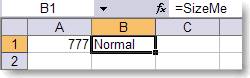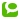Defining names in Excel is usually limited to ranges (1 cell is also a range).
Names are easy to define, and make formulas easier to read (look at the VLOOKUP post I did a couple of weeks ago).
But you can be pretty creative with names too.
Look at the following example:In A2 we got the formula:
=IF(A1>1000,"Big",IF(A1>500,"Normal","Small"))
A simple formula, but it could be way more complex.
Imagine you use this formula all over the place,
and now suppose you change your mind about the criteria for 'Big'.
You need to change every cell where you've used the formula.

There are 2 solutions:

• You create a custom function using VB or Macros
• You use a Name
Solution 1 is easy enough to do, but a little overkill.

Solution 2 is what this post is all about, and is very easy to do:
• First goto to cell A2
• Copy the formula (to the clipboard)
• Create a new name (use Insert/Name/Define):
Name: "SizeMe"
Refers To: paste your formula in here
Click OK to close the Name definition dialog box.
• Put the following formula in A2:  =SizeMeMagic, isn't it?
Now if you need to change the formula, just redefine (adjust) the formula in the name-definition dialogbox and it will recalc everywhere you have used '=SizeMe'.

Category:  Microsoft Excel  |Technorati: ,